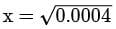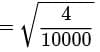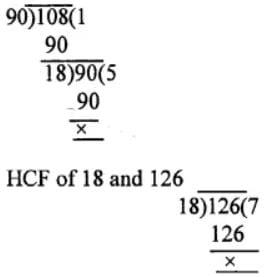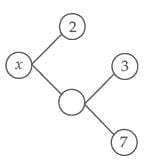# Test: Real Numbers (Hard)

## 20 Questions MCQ Test Additional Documents & Tests for Class 10 | Test: Real Numbers (Hard)

Description
Attempt Test: Real Numbers (Hard) | 20 questions in 20 minutes | Mock test for Class 10 preparation | Free important questions MCQ to study Additional Documents & Tests for Class 10 for Class 10 Exam | Download free PDF with solutions
QUESTION: 1

### Show that x is rational if: x2 = 0.0004

Solution: x2 = 0.0004= 2 / 100 = 0.02, which is rational

QUESTION: 2

### Without doing any actual division, find which of the following rational numbers have terminating decimal representation:(i) 7 / 16(ii) 23 / 125(iii) 9 / 14(iv) 32 / 45(v) 43 / 50(vi) 17 / 40(vii) 61 / 75(viii) 123 / 250

Solution: The rational not having denominator as multiple of 2m x 5n will be non terminating.

(i) 7 / 16 = 7 / 2 x 2 x 2 x 2 denominator multiple of 2m x 5n hence terminating.

(ii) 23 / 125 = 23 / 5 x 5 x 5 denominator multiple of 2m x 5n hence terminating.

(iii) 9 / 14 = 9 / 7 x 2 denominator is not multiple of 2m x 5n hence non-terminating.

(iv) 32 / 45 = 32 / 3 x 3 x 5 denominator is not multiple of 2m x 5n hence non-terminating.

(v) 43 / 50 = 43 / 5 x 5 x 2 denominator multiple of 2m x 5n hence terminating.

(vi) 17 / 40 = 17 / 2 x 2 x 2 x 5 denominator multiple of 2m x 5n hence terminating.

(vii) 61 / 75 = denominator is not multiple of 2m x 5n hence non-terminating.

(viii) 123 / 250 = 123 / 5 x 5 x 5 x 2 denominator multiple of 2m x 5n hence terminating.

(i), (ii), (v), (vi) and (viii) will have terminating decimal.

QUESTION: 3

### Prove that 3 + √5 is an irrational number.

Solution: Let us assume that √5 is a rational number which can be expressed in the form of p / q, where p and q are integers, q ≠ 0 and p and q are co prime that is HCF (p, q) = 1.
QUESTION: 4

Find the greatest number that will divide 93, 111 and 129, leaving remainder 3 in each case.

Solution: Since Remainder is 3 in each case numbers are

93 - 3 = 90

111 - 3 = 108

129 - 3 = 126

Required number will be H.C.F of 90,108 and 126

H.C.F of 90 and 108∴ The greatest number is 18.

QUESTION: 5

If the product of two numbers is 540 and their HCF is 30, find their LCM.

Solution: The product of two numbers = 540

HCF x LCM = 540

30 x LCM = 540

LCM = 540 / 30 = 18

QUESTION: 6

π is an irrational number.

Solution: π is an irrational number, which means that it is a real number that cannot be expressed by a simple fraction. That's because pi is what mathematicians call an "infinite decimal" — after the decimal point, the digits go on forever and ever. ... (These rational expressions are only accurate to a couple of decimal places.)
QUESTION: 7

The sum of two irrational numbers is an irrational number.

Solution: An irrational number is a number that cannot be expressed as a fraction for any integers and. Irrational numbers have decimal expansions that neither terminate nor become periodic.
QUESTION: 8

If the product of two co-primes is 553, then their LCM = _____

Solution: The HCF of co-prime no.s is 1

∴ Product of the two numbers = HCF × LCM

Thus LCM = 553

QUESTION: 9

The value of x in following factor tree is ____Solution: 1 × 42 = 42

2 × 21 = 42

3 × 14 = 42

6 × 7 = 42

Therefore, the factors of 42 in pairs are (1, 42), (2, 21), (3, 14) and (6, 7).

QUESTION: 10

Product of any irrational number with a rational number 'x' is always rational. Then, x is ____.

Solution: Product of any rational no. with irrational is always irrational only when irrational no. is not a perfect square and is not having 0 , 1 as roots.
QUESTION: 11

The prime factorisation of 320 is____.

Solution: 320 = 2 x 2 x 2 x 2 x 2 x 2 x 5

320 = 26 x 51

QUESTION: 12

Any number ending with '0' must have ___ and ___ as its prime factors.

Solution: For example: 10, 20, 30, .....

The factors of 10 = 1, 2, 5 and

The prime factors of 10 = 2 and 5

The factors of 20 = 1, 2, 4, 5, 10 and 20

The prime factors of 20 = 2 and 5

∴ Any number ending with '0' must have '2' and '5' as its prime factors.

QUESTION: 13

HCF of two numbers is always a factor of their LCM.

Solution: This statement HCF of two numbers is always a factor of their LCM is TRUE.

Because HCF is a factor of both the numbers which are factors of their LCM.

Hence, HCF of two numbers is always a factor of their LCM.

QUESTION: 14

Every even integer is of the form 2m, where m is an integer.

Solution: This statement every even integer is of the form 2m, where m is an integer is TRUE.

For Example : Let the value of m be -2, 4 & 0

Then the values for 2m = 2 × -2 , 2 × 4 & 2 × 0

= - 4 , 8 & 0

QUESTION: 15

If p and q are relatively prime numbers, what is their LCM?

Solution: If p and q are prime numbers, they do not have any common factor.

p = 1 × p

q = 1 × q

HCF of a and b = 1.

Their LCM = 1 × p × q

LCM of p and q = pq

QUESTION: 16

For what value of n, 2n x 5n ends in 5.

Solution: 2m × 5n can also be written as

2n × 5n = (2 × 5)n = (10)n

which always ends in zero, as 102 = 100, 103 = 1000,.....

Thus, there is no value of n for which (2n × 5n ) ends in 5.

QUESTION: 17

LCM of two prime numbers is 1.

Solution: A prime number is a natural number greater than 1 which has no divisor other than 1 and itself. The least common multiple (LCM) of two numbers is the smallest non zero number which is a multiple of both numbers. considering the L.C.M of two primes : 2 and 3 , here the L.C.M = 2 × 3 = 6.
QUESTION: 18

LCM of two or more ____ is equal to their product.

Solution: Prime numbers are numbers that have only 2 factors: 1 and themselves. For example, the first 5 prime numbers are 2, 3, 5, 7, and 11. By contrast, numbers with more than 2 factors are called composite numbers.
QUESTION: 19

If a and b are two odd prime numbers, then a2 - b2 is ____(prime/composite/neither prime nor composite).

Solution: Composite numbers can be defined as the whole numbers that have more than two factors. Whole numbers that are not prime are composite numbers, because they are divisible by more than two numbers.
QUESTION: 20

HCF of 870 and 225 is:

Solution: Factors of 870 = 5 × 2 × 3 × 29

Factors of 225 = 5 × 5 × 3 × 3

Common factors = 5 × 3 = 15

H.C.F = 15Use Code STAYHOME200 and get INR 200 additional OFF Use Coupon Code